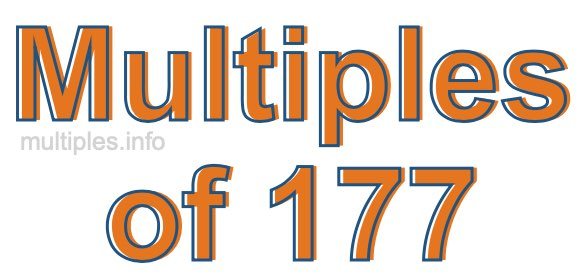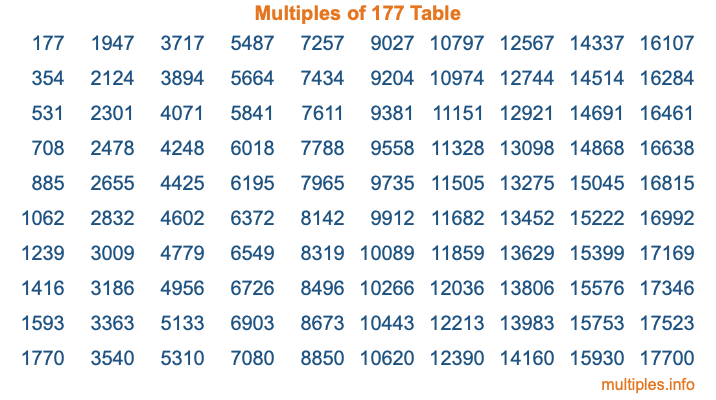Multiples of 177Welcome to the Multiples of 177 page. Here we will first teach you everything you will ever need to know about the multiples of 177, and then give you a study guide summary of everything we taught you to make sure you remember it all. Use this page to look up facts and learn information about the multiples of 177. This page will make you a multiples of one hundred seventy-seven expert!

Definition of Multiples of 177
Multiples of 177 are all the numbers that when divided by 177 equal an integer. Each of the multiples of 177 are called a multiple. A multiple of 177 is created by multiplying 177 by an integer.

Therefore, to create a list of multiples of 177, you start with 1 multiplied by 177, then 2 multiplied by 177, then 3 multiplied by 177, and so on for as long as you want. Thus, the list of the first five multiples of 177 is 177, 354, 531, 708, and 885. To see a larger list of multiples of 177, see the printable image of Multiples of 177 further down on this page. We also have a category where you can choose any nth multiple of 177.

Multiples of 177 Checker
The Multiples of 177 Checker below checks to see if any number of your choice is a multiple of 177. In other words, it checks to see if there is any number (integer) that when multiplied by 177 will equal your number. To do that, we divide your number by 177. If the the quotient is an integer, then your number is a multiple of 177.

Is  a multiple of 177?

Least Common Multiple of 177 and ...
A Least Common Multiple (LCM) is the lowest multiple that two or more numbers have in common. This is also called the smallest common multiple or lowest common multiple and is useful to know when you are adding our subtracting fractions. Enter one or more numbers below (177 is already entered) to find the LCM.

Check out our LCM Calculator if you need more details about the Least Common Multiple or if you need the LCM for different numbers for adding and subtraction fractions.

nth Multiple of 177
As we stated above, 177 is the first multiple of 177, 354 is the second multiple of 177, 531 is the third multiple of 177, and so on. Enter a number below to find the nth multiple of 177.

th multiple of 177

Multiples of 177 vs Factors of 177
177 is a multiple of 177 and a factor of 177, but that is where the similarities end. All postive multiples of 177 are 177 or greater than 177. All positive factors of 177 are 177 or less than 177.

Below is the beginning list of multiples of 177 and the factors of 177 so you can compare:

Multiples of 177: 177, 354, 531, 708, 885, etc.

Factors of 177: 1, 3, 59, 177

As you can see, the multiples of 177 are all the numbers that you can divide by 177 to get a whole number. The factors of 177, on the other hand, are all the whole numbers that you can multiply by another whole number to get 177.

It's also interesting to note that if a number (x) is a factor of 177, then 177 will also be a multiple of that number (x).

Multiples of 177 vs Divisors of 177
The divisors of 177 are all the integers that 177 can be divided by evenly. Below is a list of the divisors of 177.

Divisors of 177: 1, 3, 59, 177

The interesting thing to note here is that if you take any multiple of 177 and divide it by a divisor of 177, you will see that the quotient is an integer.

Multiples of 177 Table
Below is an image of the first 100 multiples of 177 in a table. The table is in chronological order, column by column. The first column has the first ten multiples of 177, the second column has the next ten multiples of 177, and so on.The Multiples of 177 Table is also referred to as the 177 Times Table or Times Table of 177. You are welcome to print out our table for your studies.

Negative Multiples of 177
Although not often discussed or needed in math, it is worth mentioning that you can make a list of negative multiples of 177 by multiplying 177 by -1, then by -2, then by -3, and so on, to get the following list of negative multiples of 177:

-177, -354, -531, -708, -885, etc.

Multiples of 177 Summary
Below is a summary of important Multiples of 177 facts that we have discussed on this page. To retain the knowledge on this page, we recommend that you read through the summary and explain to yourself or a study partner why they hold true.

There are an infinite number of multiples of 177.

A multiple of 177 divided by 177 will equal a whole number.

177 divided by a factor of 177 equals a divisor of 177.

The nth multiple of 177 is n times 177.

The largest factor of 177 is equal to the first positive multiple of 177.

177 is a multiple of every factor of 177.

177 is a multiple of 177.

A multiple of 177 divided by a divisor of 177 equals an integer.

177 divided by a divisor of 177 equals a factor of 177.

Any integer times 177 will equal a multiple of 177.

Multiples of a Number
Here you can get the multiples of another number, all with the same attention to detail as we did for multiples of 177 on this page.

Multiples of
Multiples of 178
Did you find our page about multiples of one hundred seventy-seven educational? Do you want more knowledge? Check out the multiples of the next number on our list!